JEE  >  NCERT Solutions Exercise 5.7: Continuity & Differentiability

# NCERT Solutions Exercise 5.7: Continuity & Differentiability - Mathematics (Maths) for JEE Main & Advanced

Continuity & Differentiability

Question 1: Find the second order derivatives of the function.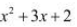Let y =Then,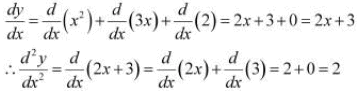Question 2: Find the second order derivatives of the function. x20

Let y = x20
Then,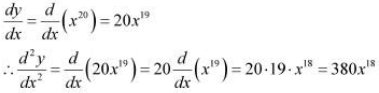Question 3: Find the second order derivatives of the function. x.cos x

Let y = x.cos x
Then,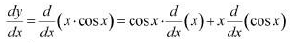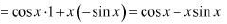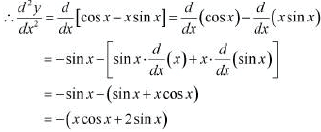Question 4: Find the second order derivatives of the function. log x

Let y = log x
Then,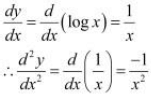Question 5: Find the second order derivatives of the function. x3 log x

Let y = x3 log x
Then,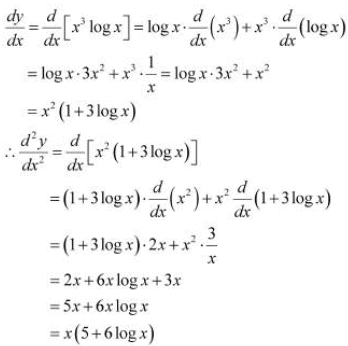Question 6: Find the second order derivatives of the function.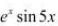Let y =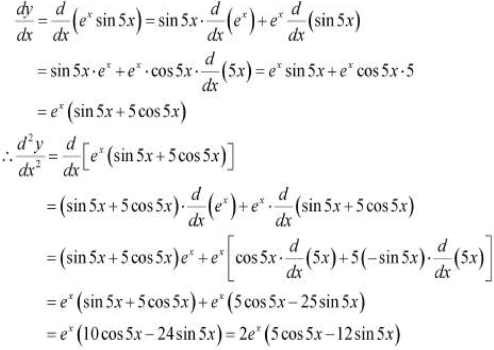Question 7: Find the second order derivatives of the function.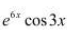Let y =Then,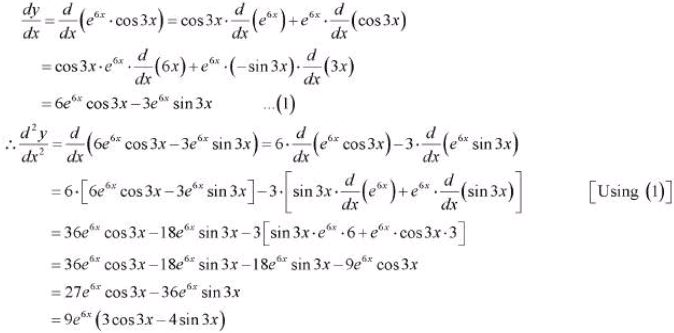Question 8: Find the second order derivatives of the function.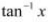Let y =Then,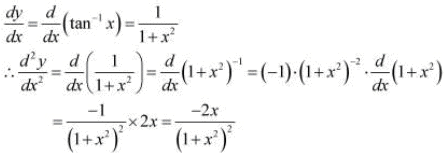Question 9: Find the second order derivatives of the function.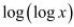Let y =Then,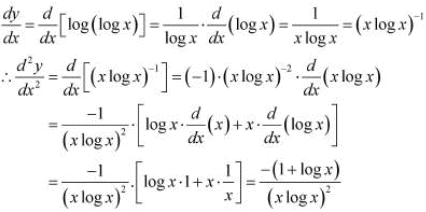Question 10: Find the second order derivatives of the function.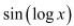Let y =Then,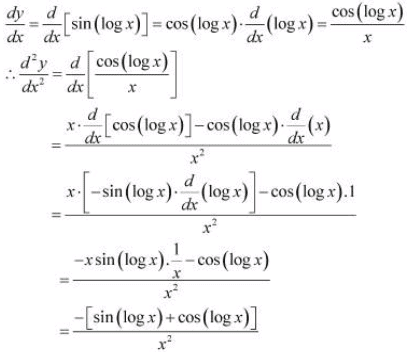Question 11: If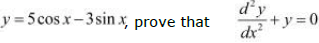It is given that,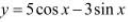Then,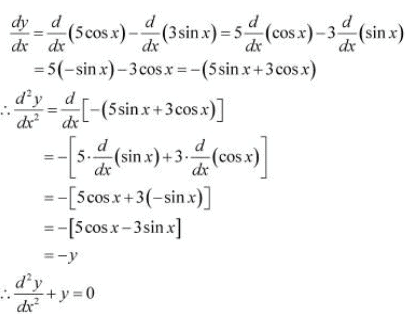Hence, proved.

Question 12:
If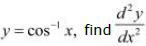in terms of y alone.

It is given that,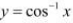Then,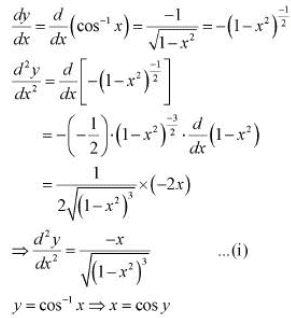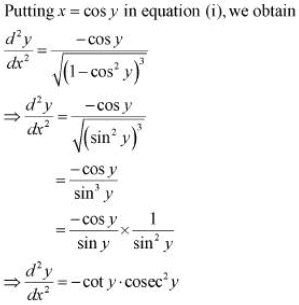Question 13:
If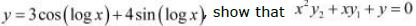It is given that,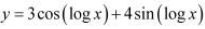Then,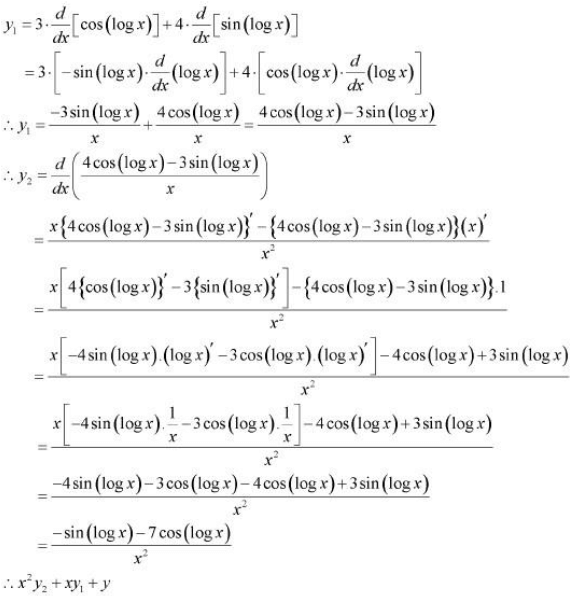Hence, proved.

Question 14:
If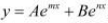, show that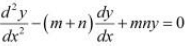It is given that,Then,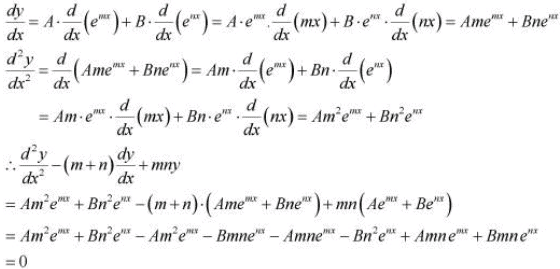Hence, proved.

Question 15:
If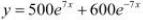, show that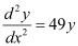It is given that,Then,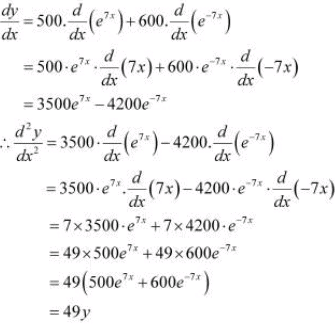Hence, proved.

Question 16:
If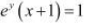, show that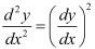The given relationship is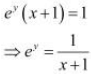Taking logarithm on both the sides, we obtain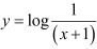Differentiating this relationship with respect to x, we obtain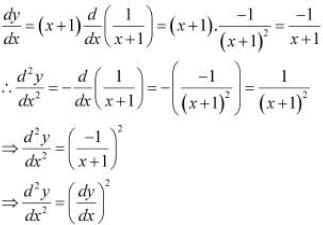Hence, proved.

Question 17:
If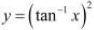, show that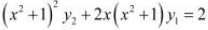The given relationship isThen,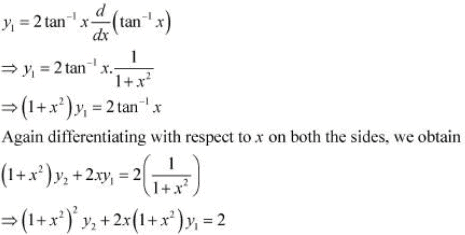Hence, proved.

The document NCERT Solutions Exercise 5.7: Continuity & Differentiability | Mathematics (Maths) for JEE Main & Advanced is a part of the JEE Course Mathematics (Maths) for JEE Main & Advanced.
All you need of JEE at this link: JEE

## FAQs on NCERT Solutions Exercise 5.7: Continuity & Differentiability - Mathematics (Maths) for JEE Main & Advanced

 1. What is continuity in calculus?Ans. Continuity in calculus refers to the property of a function where there are no abrupt jumps, holes, or vertical asymptotes. In simpler terms, a function is continuous if its graph can be drawn without lifting the pencil from the paper. Continuity is a fundamental concept in calculus and is essential for various applications in mathematics and science.
 2. What is differentiability in calculus?Ans. Differentiability in calculus refers to the property of a function where it has a derivative at every point within its domain. A function is said to be differentiable if the rate of change of the function is well-defined at each point. Differentiability is closely related to continuity, as a function must be continuous in order to be differentiable.
 3. How do you determine if a function is continuous?Ans. To determine if a function is continuous, we need to check three conditions: 1) The function must be defined at the given point. 2) The limit of the function as it approaches the given point must exist. 3) The value of the function at the given point must be equal to the limit. If all three conditions are satisfied, then the function is continuous at that point. However, if any of the conditions fail, then the function is discontinuous at that point.
 4. What is the difference between continuity and differentiability?Ans. Continuity and differentiability are related but distinct concepts in calculus. - Continuity refers to the smoothness of a function's graph without any abrupt jumps or holes. A function can be continuous without being differentiable. - Differentiability refers to the existence of a derivative at each point within the function's domain. A function must be continuous in order to be differentiable, but not all continuous functions are differentiable. In simpler terms, continuity focuses on the behavior of the function's graph, while differentiability focuses on the rate of change of the function.
 5. What are the applications of continuity and differentiability in real life?Ans. Continuity and differentiability have various applications in real-life scenarios, such as: - Physics: Continuity and differentiability concepts are utilized in modeling physical phenomena, such as motion, fluid flow, and electrical circuits. - Economics: These concepts are used to analyze and optimize economic models, such as supply and demand functions, production functions, and cost functions. - Engineering: Continuity and differentiability are essential in engineering fields, such as civil engineering, mechanical engineering, and electrical engineering, for analyzing and designing structures, systems, and processes. - Medicine: Continuity and differentiability concepts are applied in medical research and data analysis for studying physiological processes, drug concentration levels, and disease progression models.

## Mathematics (Maths) for JEE Main & Advanced

129 videos|359 docs|306 tests

## Mathematics (Maths) for JEE Main & Advanced

129 videos|359 docs|306 tests
Signup to see your scores go up within 7 days! Learn & Practice with 1000+ FREE Notes, Videos & Tests.
10M+ students study on EduRev
Track your progress, build streaks, highlight & save important lessons and more!(Scan QR code)
Related Searches

,

,

,

,

,

,

,

,

,

,

,

,

,

,

,

,

,

,

,

,

,

;